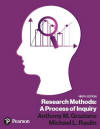Graziano & Raulin
Research Methods (9th edition)

## WEB Links to Topics Covered in Chapter 5

Listed below are several links to Web sites that include information about topics covered in this chapter. We will be periodically updating this list to keep it fresh and interesting. If you know of a good site on a topic of relevance to this chapter, we would appreciate hearing about it. Contact Mike Raulin with your suggestions. We will be sure to give you credit for suggesting high quality sites in future updates.

### Statistics Resources

Statistical Resources on the Web
A list of statistical resources compiled by Michael Friendly of York University.

Electronic Textbook on Statistics
Excellent resource, but tends to load very slowly. You may want to avoid this site unless you have a high-speed connection.

Other Beginning Statistics Resources Around the WWW
An unusually extensive listing of useful sites.

Probability and Statistics
Resources for the classroom teacher. Some excellent explanations of difficult concepts.

Sydney Tyrrell's Resource Page
A collection of links to resources on statistical principles.

Intro Stats Demonstrations
Several demonstrations of some basic statistics concepts.

Psychological Statistics
A course outline, with numerous handouts on various topics.

### Path Analysis

Path Analysis
A relatively detailed discussion of path analysis.

Description of Path Analysis
This is a readable description of the process of path analysis.

Structured Equation Modeling and Path Analysis
This is a 10-page description of these methods.

### Statistical Regression

Introduction to Regression Analysis
Descriptions of the logic and mathematics of regression analysis.

How to Read the Output From Simple Linear Regression Analyses
The title says it all.

Demonstration of Regression
(requires a JAVA-enabled browser)

Computing Regression Equations
This site does the computation of a both simple and multiple regression for small data sets. It is a nice way to see how the models respond to one or two deviant scores.

Regression analysis
A mathematically detailed discussion of regression.

Introduction to Multiple Regression
Description of this extension of simple regression by Hyperstat Online Contents.

SPSS Regression Models
Discusses the many types of regression analyses that can be conducted with SPSS.

### Analysis of Variance (ANOVA)

One-Way ANOVA
Detailed description of the one-way ANOVA.

Two-Way ANOVA
Detailed description of the two-way ANOVA.

Understanding ANOVA Visually
This hands-on site allows you to see how group differences affect the ANOVA computations. Great for giving you a conceptual feel for this complex procedure.

HyperStat Online's Introduction to ANOVA
A fairly detailed discussion of ANOVA and its related topics.

SPSS Topics: ANOVA
Discusses extensively the issues surrounding ANOVA.

Power Analysis for ANOVA Designs
This site not only discusses the issue of power in ANOVA designs, but also has a little program for computing power.

### Analysis of Covariance (ANCOVA)

Description of ANCOVA
This is a brief description of this procedure.

A New View of Statistics
Discussion of both ANOVA and ANCOVA models (Note: high level).

Statistical Analysis of the Analysis of Covariance Design
Discusses the mathematical underpinnings of this approach.

Covariance Designs
Description of the principles behind ANCOVA, with liberal use of scatter plots and regression lines.